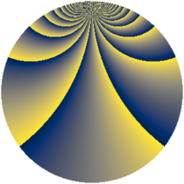# Properties

 Label 2880.2.dkLevel $2880$ Weight $2$ Character orbit 2880.dk Rep. character $\chi_{2880}(257,\cdot)$ Character field $\Q(\zeta_{12})$ Dimension $560$ Sturm bound $1152$

# Learn more about

## Defining parameters

 Level: $$N$$ $$=$$ $$2880 = 2^{6} \cdot 3^{2} \cdot 5$$ Weight: $$k$$ $$=$$ $$2$$ Character orbit: $$[\chi]$$ $$=$$ 2880.dk (of order $$12$$ and degree $$4$$) Character conductor: $$\operatorname{cond}(\chi)$$ $$=$$ $$45$$ Character field: $$\Q(\zeta_{12})$$ Sturm bound: $$1152$$

## Dimensions

The following table gives the dimensions of various subspaces of $$M_{2}(2880, [\chi])$$.

Total New Old
Modular forms 2400 592 1808
Cusp forms 2208 560 1648
Eisenstein series 192 32 160

## Trace form

 $$560q + 12q^{5} + O(q^{10})$$ $$560q + 12q^{5} + 4q^{13} + 16q^{21} - 4q^{25} - 20q^{33} + 16q^{37} - 24q^{41} - 12q^{45} + 16q^{57} + 8q^{61} - 12q^{65} - 16q^{73} + 12q^{77} + 16q^{81} + 4q^{85} + 92q^{93} - 4q^{97} + O(q^{100})$$

## Decomposition of $$S_{2}^{\mathrm{new}}(2880, [\chi])$$ into newform subspaces

The newforms in this space have not yet been added to the LMFDB.

## Decomposition of $$S_{2}^{\mathrm{old}}(2880, [\chi])$$ into lower level spaces

$$S_{2}^{\mathrm{old}}(2880, [\chi]) \cong$$ $$S_{2}^{\mathrm{new}}(45, [\chi])$$$$^{\oplus 7}$$$$\oplus$$$$S_{2}^{\mathrm{new}}(90, [\chi])$$$$^{\oplus 6}$$$$\oplus$$$$S_{2}^{\mathrm{new}}(180, [\chi])$$$$^{\oplus 5}$$$$\oplus$$$$S_{2}^{\mathrm{new}}(360, [\chi])$$$$^{\oplus 4}$$$$\oplus$$$$S_{2}^{\mathrm{new}}(720, [\chi])$$$$^{\oplus 3}$$$$\oplus$$$$S_{2}^{\mathrm{new}}(1440, [\chi])$$$$^{\oplus 2}$$